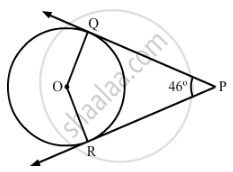# n Fig. 2, PQ and PR are two tangents to a circle with centre O. If ∠QPR = 46°, then ∠QOR equals - Mathematics

n Fig. 2, PQ and PR are two tangents to a circle with centre O. If ∠QPR = 46°, then ∠QOR equals:(A) 67°
(B) 134°
(C) 44°
(D) 46°

#### Solution

Given: ∠QPR = 46°
PQ and PR are tangents.
Therefore, the radius drawn to these tangents will be perpendicular to the tangents.
So, we have OQ ⊥ PQ and OR ⊥ RP.
⇒ ∠OQP = ∠ORP = 90
So, in quadrilateral PQOR, we have
∠OQP +∠QPR + ∠PRO + ∠ROQ = 360
⇒ 90° + 46° + 90° + ∠ROQ = 360
⇒ ∠ROQ = 360 − 226 = 134

Hence, the correct option is B.

Concept: Concept of Circle - Centre, Radius, Diameter, Arc, Sector, Chord, Segment, Semicircle, Circumference, Interior and Exterior, Concentric Circles
Is there an error in this question or solution?